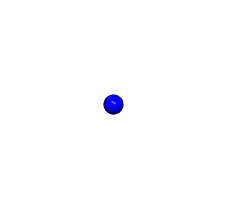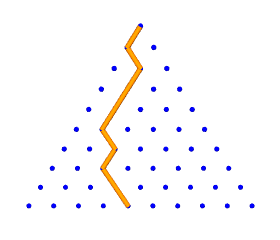# Crystal Ball Sequence¶

The face-centered cubic (FCC) lattice is not always presented in this simplest form, ditto the cubic close packing (CCP), which amounts to the same thing. A nuclear ball is surrounded by a layer of twelve, all touching it, and adjacent neighbors. The shape so formed is not a cube, but a cuboctahedron, with eight triangular faces and six square. This is where I can type stuff.

As the cuboctahedral packing continues to expand outward, layer by layer, the cumulative number of balls or points forms the Crystal Ball Sequence.

cubocta(), a generator, yields the number of balls in each successive layer of the cuboctahedron, according to a simple formula derived by R. Buckminster Fuller, a prolific inventor and philosopher . cummulative( ) delegates to cubocta( ) while accumulating the number in each layer to provide a running total.In :
from itertools import accumulate, islice

def cubocta():
"""
Classic Generator:  Cuboctahedral / Icosahedral #s
https://oeis.org/A005901
"""
yield 1  # nuclear ball
f = 1
while True:
elem = 10 * f * f + 2  # f for frequency
yield elem    # <--- pause / resume here
f += 1

def cummulative(n):
"""
https://oeis.org/A005902 (crystal ball sequence)
"""
yield from islice(accumulate(cubocta()),0,n)

print("{:=^30}".format(" Crystal Ball Sequence "))
print("{:^10} {:^10}".format("Layers", "Points"))
for f, out in enumerate(cummulative(30),start=1):
print("{:>10} {:>10}".format(f, out))


=== Crystal Ball Sequence ====
Layers     Points
1          1
2         13
3         55
4        147
5        309
6        561
7        923
8       1415
9       2057
10       2869
11       3871
12       5083
13       6525
14       8217
15      10179
16      12431
17      14993
18      17885
19      21127
20      24739
21      28741
22      33153
23      37995
24      43287
25      49049
26      55301
27      62063
28      69355
29      77197
30      85609


# Octet Truss¶

When adjacent CCP ball centers interconnect, what do you get? Why the octet truss of course, a well known space frame, used a lot in architecture. Alexander Graham Bell was fascinated by this construction.

 Siobahn Roberts. King of Infinite Space. New York: Walker & Company (2006). pp 179-180.

"Coxeter sent back a letter saying that one equation would be 'a remarkable discovery, justifying Bucky's evident pride,' if only it weren't too good to be true. The next day, Coxeter called: 'On further reflection, I see that it is true'. Coxeter told Fuller how impressed he was with his formula -- on the cubic close-packing of balls."

 http://worldgame.blogspot.com/2006/02/octet-truss.html (additional info on the octet truss)

# Pascal's Triangle¶

Pascal's Triangle connects to the Binomial Theorem (originally proved by Sir Isaac Newton) and to numerous topics in probability theory. The triangular and tetrahedral number sequences may be discovered lurking in its columns.

pascal(), a generator, yields successive rows of Pascal's Triangle. By prepending and appending a zero element and adding vertically, a next row is obtained. For example, from  we get [0, 1] + [1, 0] = [1, 1]. From [1, 1] we get [0, 1, 1] + [1, 1, 0] = [1, 2, 1] and so on.

Notice the triangular numbers (1, 3, 6, 10...) and tetrahedral number sequences (1, 4, 10, 20...) appear in the slanted columns.In :
from itertools import islice

def pascal():
row = 
while True:
yield row
row = [i+j for i,j in zip(+row, row+)]

print("{0:=^60}".format(" Pascal's Triangle "))
print()
for r in islice(pascal(),0,11):
print("{:^60}".format("".join(map(lambda n: "{:>5}".format(n), r))))

==================== Pascal's Triangle =====================

1
1    1
1    2    1
1    3    3    1
1    4    6    4    1
1    5   10   10    5    1
1    6   15   20   15    6    1
1    7   21   35   35   21    7    1
1    8   28   56   70   56   28    8    1
1    9   36   84  126  126   84   36    9    1
1   10   45  120  210  252  210  120   45   10    1


Each number in Pascal's Triangle may be understood as the number of unique pathways to that position, were falling balls introduced through the top and allowed to fall left or right to the next row down. This apparatus is sometimes called a Galton Board.

For example, a ball could reach the 6 in the middle of the 5th row going 1,1,2,3,6 in four ways (counting left and right mirrors), or 1,1,1,3,6 in two ways. The likely pattern when many balls fall through this maze will be a bell curve, as shown in the simulation below.

In :
from IPython.display import YouTubeVideo